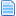# Helper 1 frame delay study & Modifying Vans' Tiny Buffer to have no delay.  (Read 1961 times)

Started by ink, August 13, 2019, 02:53:08 pm

#### ink##### Helper 1 frame delay study & Modifying Vans' Tiny Buffer to have no delay.
New #1  August 13, 2019, 02:53:08 pm
First the study
M.U.G.E.N 1.0+ only
I did a big study on the cond() exploit, helpers and the 1 tick delay. There are a good amount of limitations in general worth noting.

These two pieces of code where put in the root(statedef -1) and a helper that had keyctrl =1. When "start" is pressed, mugen pauses and the results can be viewed with debug.
Code:
`;Statedef -1[state -1]type = nulltrigger1= command = "start"trigger1 = Cond(NumHelper(33333333), Helper(33333333), Cond(1, Var(8) := 1, 0), 0) [state -1]type = nulltrigger1= command = "start"trigger1 = Cond(NumHelper(33333333), Helper(33333333), Cond(1, Var(12) := 1, 0), 0)[state -1]type = DisplayToClipboardtrigger1 = 1text = " H-Var(8) = %d, H-Var(9) = %d, H-Var(10) = %d, H-Var(11) = %d, H-Var(12) = %d  \n"params = helper(33333333),var(8) ,    helper(33333333),var(9) ,    helper(33333333),var(10),    helper(33333333),var(11),    helper(33333333),var(12)[state -1]type = pause;trigger1 = command = "start"trigger1 = helper(33333333),var(8) = 1 time = 100`

Code:
`;Helper(33333333)[state test]type = nulltrigger1 = var(8) = 1 ;acts as "start", set in root.trigger1 = var(9):= 1[state test]type = nulltrigger1 = command = "start"trigger1 = var(10):= 1[state test]type = nulltrigger1 = root, command = "start"trigger1 = var(11):= 1;var(12) is set in the root and also read in the root.`

What this code proves:
• You can set a var in a helper from the root and read it from the root with no lag. We already knew that one. ref var(8) and var(12)
• If a cond() exploit is used in the root to set a var in a helper, and that var is then used to set a second var in the helper, the root will NOT read the second helper var until 1 tick later. ref var(9)
• If keyctrl =1 for the helper and a command sets a helper var, the root will NOT read the var until 1 tick later. ref var(10)
• If redirect root, command is used in a helper to set a helper var, it will have the same results as keyctrl above. The root will NOT read the var until 1 tick later. ref var(11)

Modifying Vans' Tiny Buffer to have no delay.
M.U.G.E.N 1.0+ only
I recommend doing Vans' Tiny Buffer as you normally would and use this information to convert it afterwards(if the 1frame delay bothers you). This is to avoid a 1 frame delay. psuedocode below should help you get an understanding of how this works.
Quote
Psuedocode
-----------------
-have a helper with keyctrl = 1 ; It's best to use the same helper that is used for the buffer system. That way no redirects will need changed in the cmd file.
-in root(statedef -2) use helper(##),command = " " for triggers; this is because the root can read helper,commands with 0 lag.
-set var() in helper using cond exploit from the root(statedef -2). This allows you to set vars and read them immediately with 0 lag.
-have root(statedef -1) read helper,var() in place of commands. ; this should already be set up from using Vans' Tiny Buffer.

The idea here is to use the helper to store the vars, while executing the code and reading the helper vars from the root.

To convert Vans' Tiny Buffer all varset and varadd sctrls(in the helper) must be moved to statedef -2 and rewritten in the form of a null sctrl using the cond() exploit. Note,sysvars can not be set using cond()exploit, if any sysvars are used they can remain in the helper. *It’s wise to note what code is from Vans’ buffer system to avoid confusion in the future. I personally copy an paste his whole header and notes.

Example:
Code:
`[State 654654, VarSet]type = VarSettrigger1 = root,movehit = 2trigger2 = root,hitpausetime = 0fvar(11) = 0`
Once converted the code above becomes this.
Code:
`[State -2, VarSet]type = Nulltrigger1 = movehit = 2trigger1 = Cond(NumHelper(10371), Helper(10371), Cond(1, FVar(11) := 0, 0), 0) || 1trigger2 = hitpausetime = 0trigger2 = Cond(NumHelper(10371), Helper(10371), Cond(1, FVar(11) := 0, 0), 0) || 1`
Last Edit: October 26, 2019, 04:47:35 am by ink